Math 316 Notes and Summaries This page requires Mozilla/Firefox/Netscape 7+ or Internet Explorer 6+ MathPlayer .

Martin Flashman's Courses
MATH 316 Real Analysis I Spring, 2013
Class Notes and Summaries
Week 10 3-25 through 3-25, 2013

3-25 Listed Main properties of the definite integral that we will prove:
Included: Monotonicity. Linearity. Additivity. Bounded Constraint. Continuity of Integral Function for integrable functions. Continuous Functions are integrable. Fundamental Theorem (Derivative form) for Continuous Functions. FTofC (Evaluation form) for Continuous Functions. Mean Value Theorem for Integrals for Continuous Functions.

3-26. Additivity. Continuity of Integral Function for Integrable Functions. Continuous Functions are Integrable.

Excerpt from Spivak, Calculus, Ch 13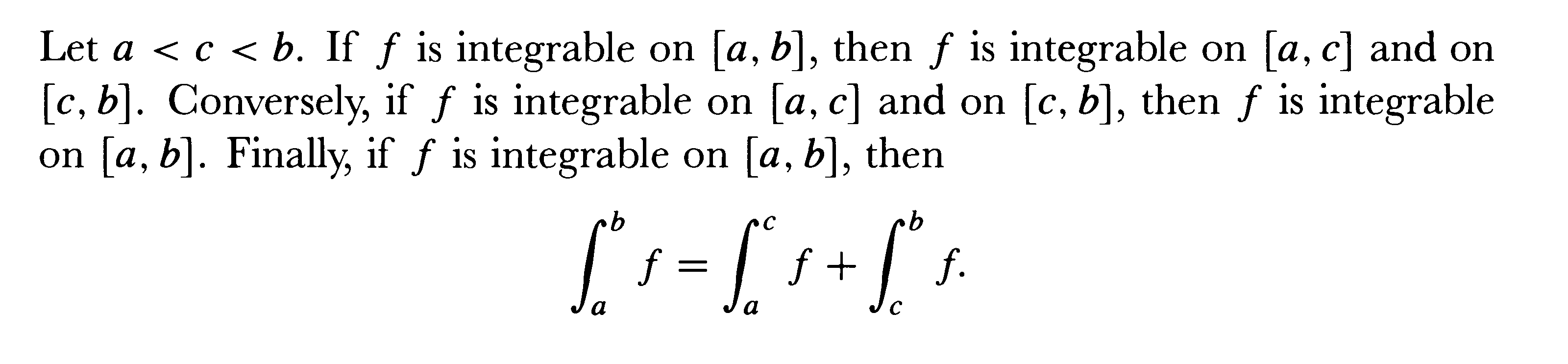Proof: [Converse proof omitted]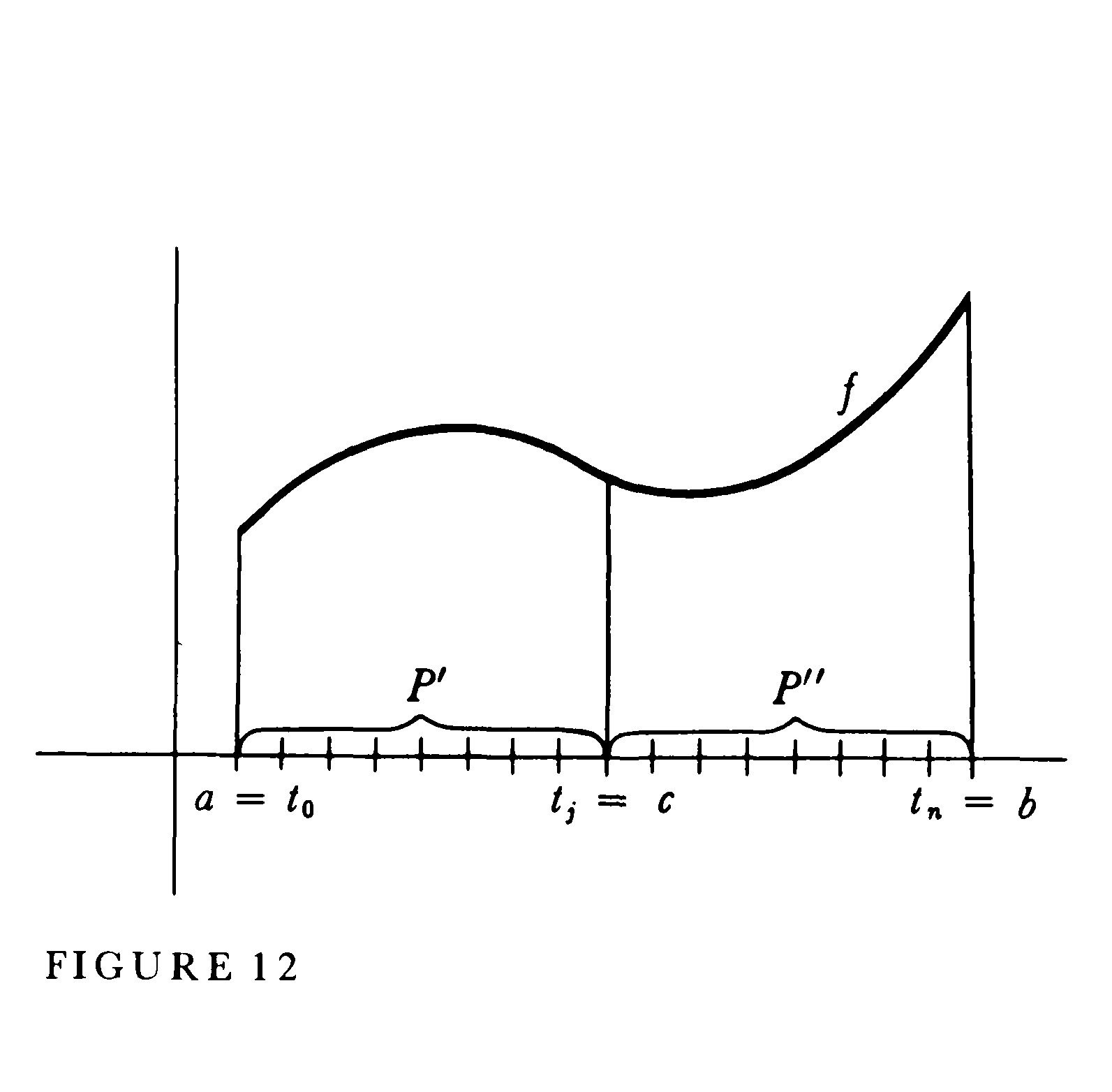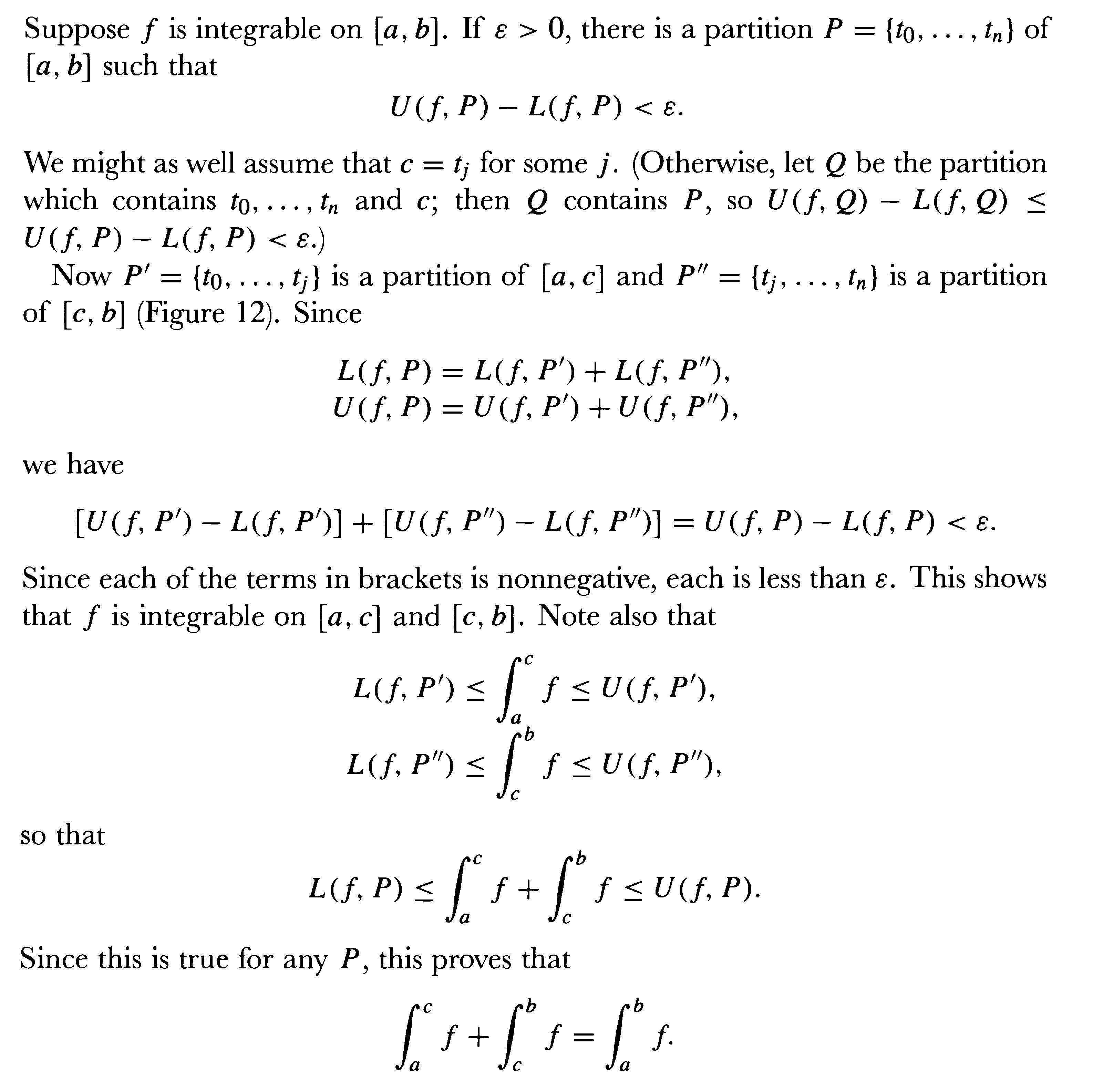EOP

Theorem: Suppose f  is integrable on [a,b] and there exist m and M so that for all x in [a,b] m \le f(x) \le M. Then m(b-a) \le \int_a^b f \le M(b-a).

Proof: Consider any partition P of [a,b], then m(b-a) \le L(f,P) and U(f,P) \le M(b-a).
Now since f is integrable,
m(b-a) \le L(f,P) \le lub {L(f,P)} =  \int_a^b f = glb{U(f,P)} \le U(f,P) \le M(b-a).  EOP

Excerpted from Spivak, Calculus, Chapter 13:

Theorem: If f  is integrable on [a,b] and  F(x) = \int_a^x f  for x in [a,b] then f is continuous on [a,b].

Proof:
Since f is bounded on the interval [a,b], we have a number M >0 so that for all x in [a,b],  -M \le f(x) \le M.
Now consider F on the interval [c, c+h], of length h>0.
Then
F(c+h) = \int_a^{c+h} f  and using additivity we have F(c+h) - F(c) = \int_c^{c+h} f .
By the previous result we have
-Mh \le  \int_c^{c+h}f  \le Mh  or
(1)    -Mh \le  F(c+h) - F(c)  \le Mh

Now consider F on the interval [c+h, c], where h<0, and length -h>0.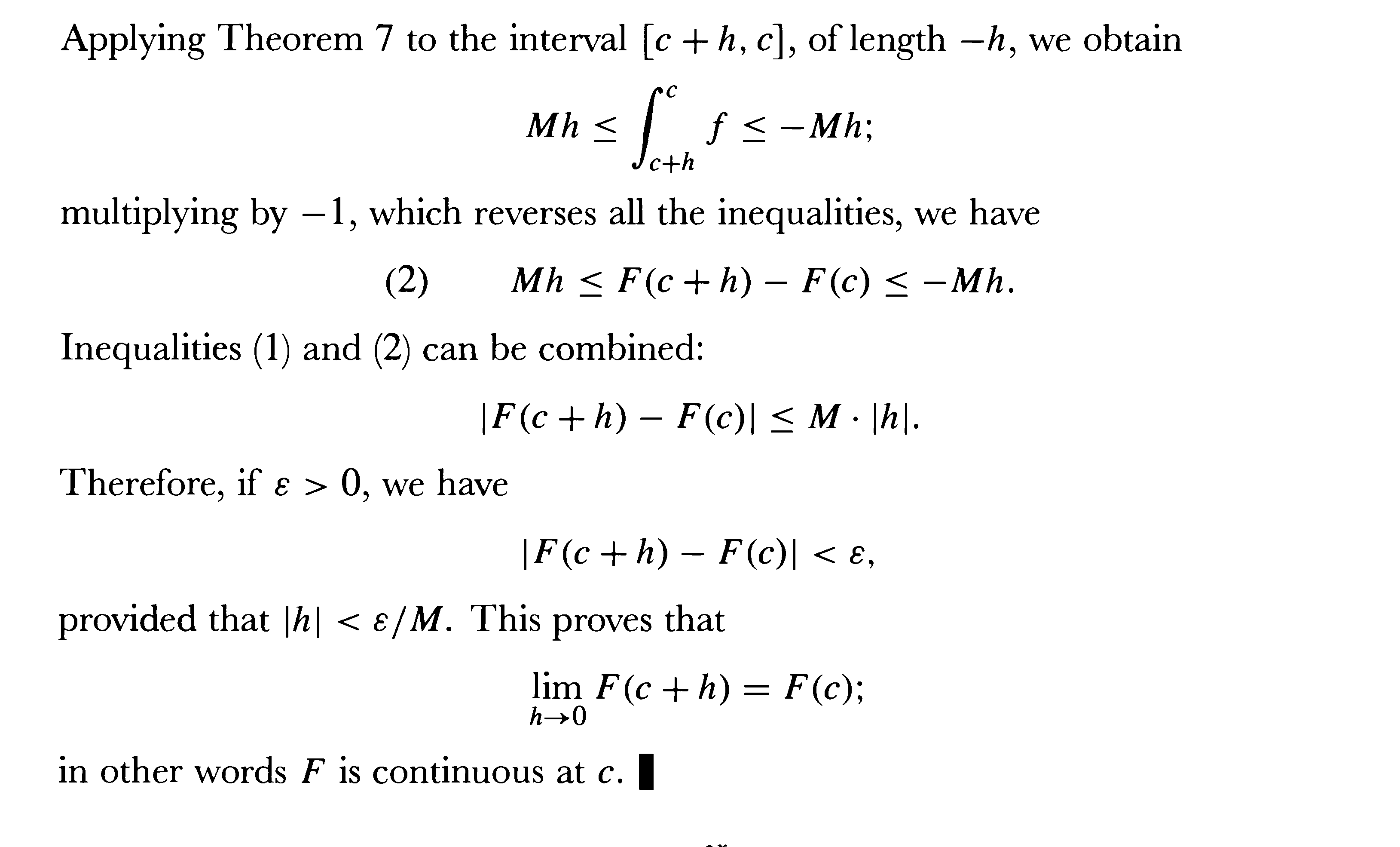3-28. FTof Calc I and II, MVT for Integrals. Alternative proof of FTofC using MVT for Integrals.

Excerpted from Spivak, Calculus, Chapter 14: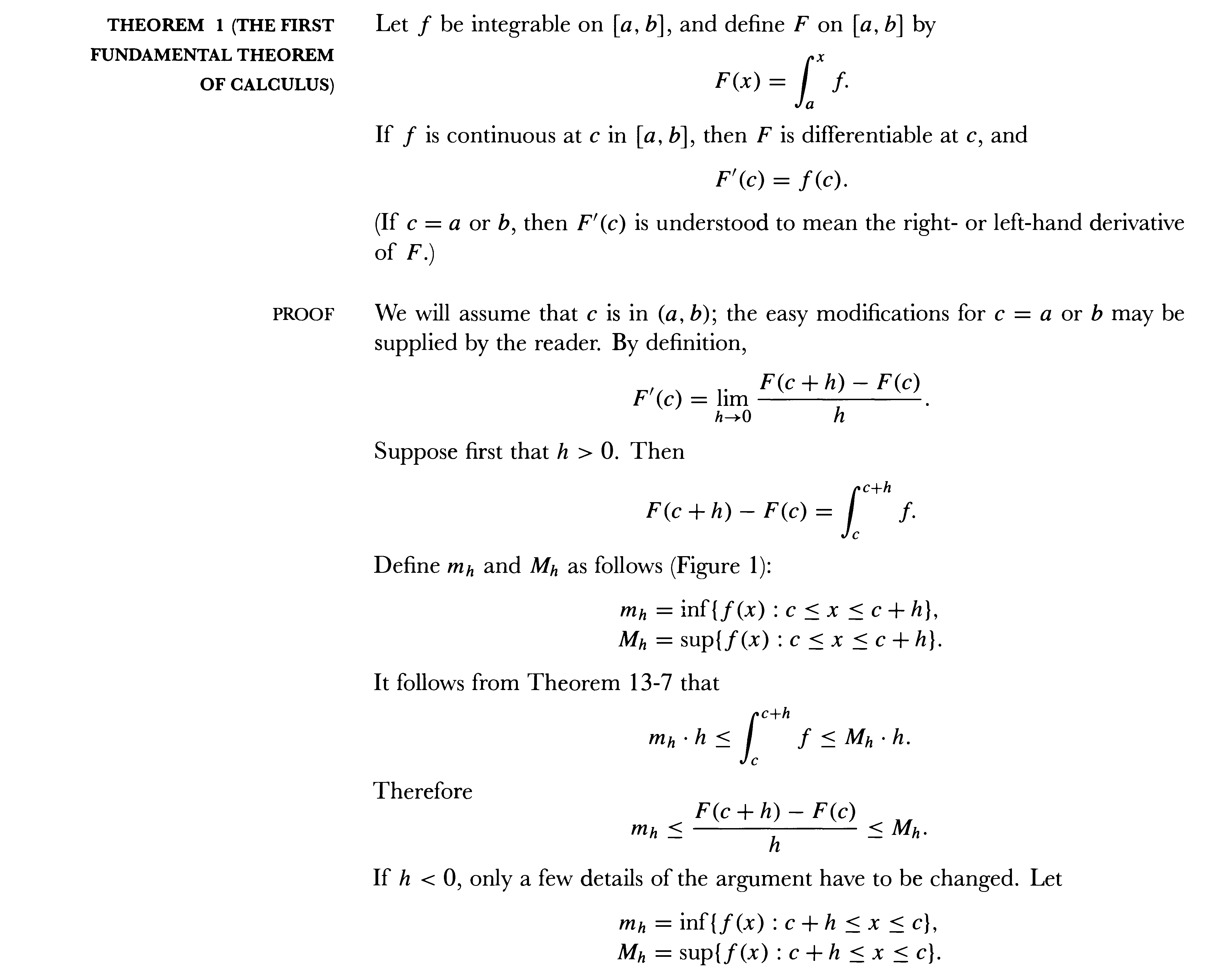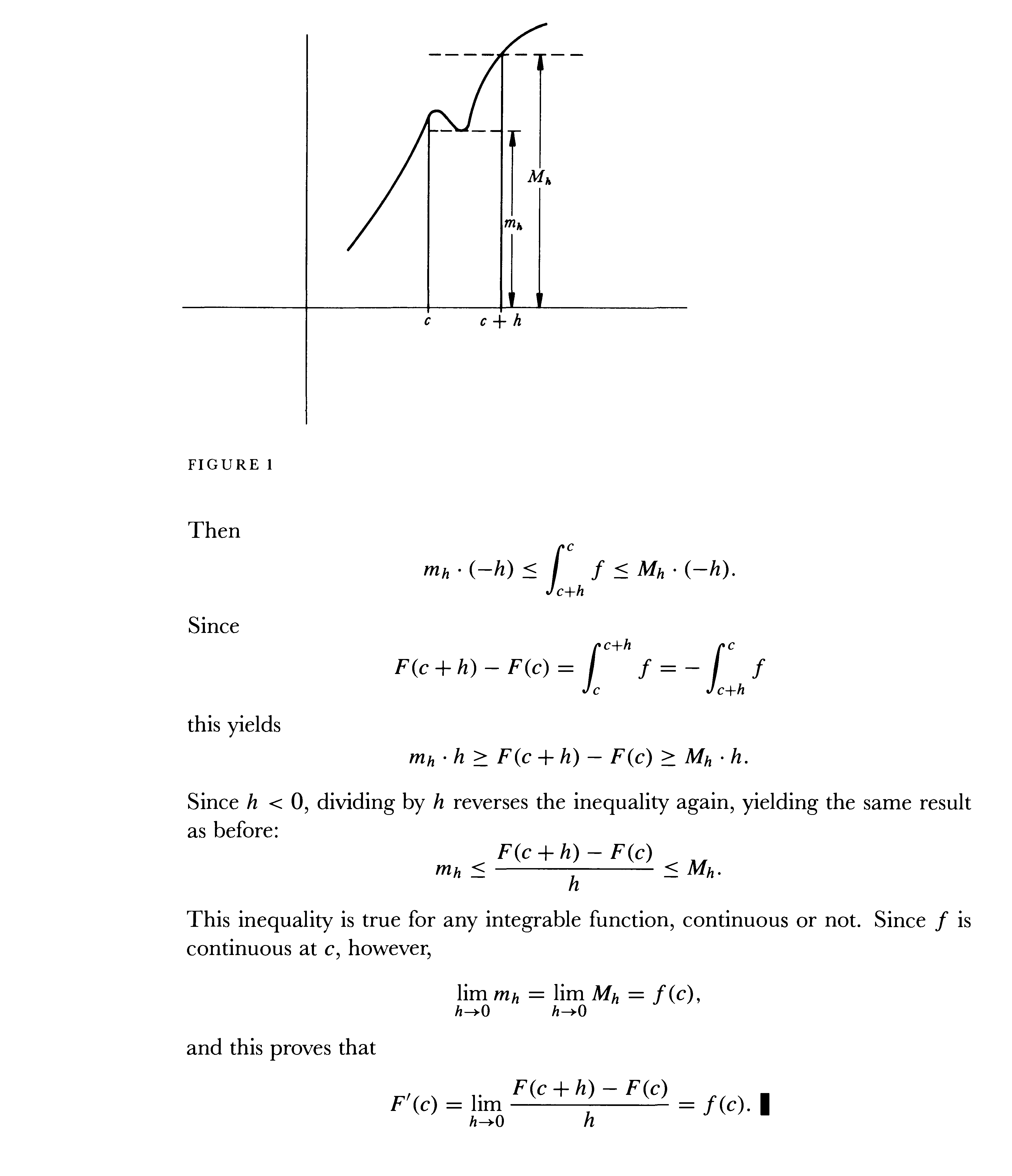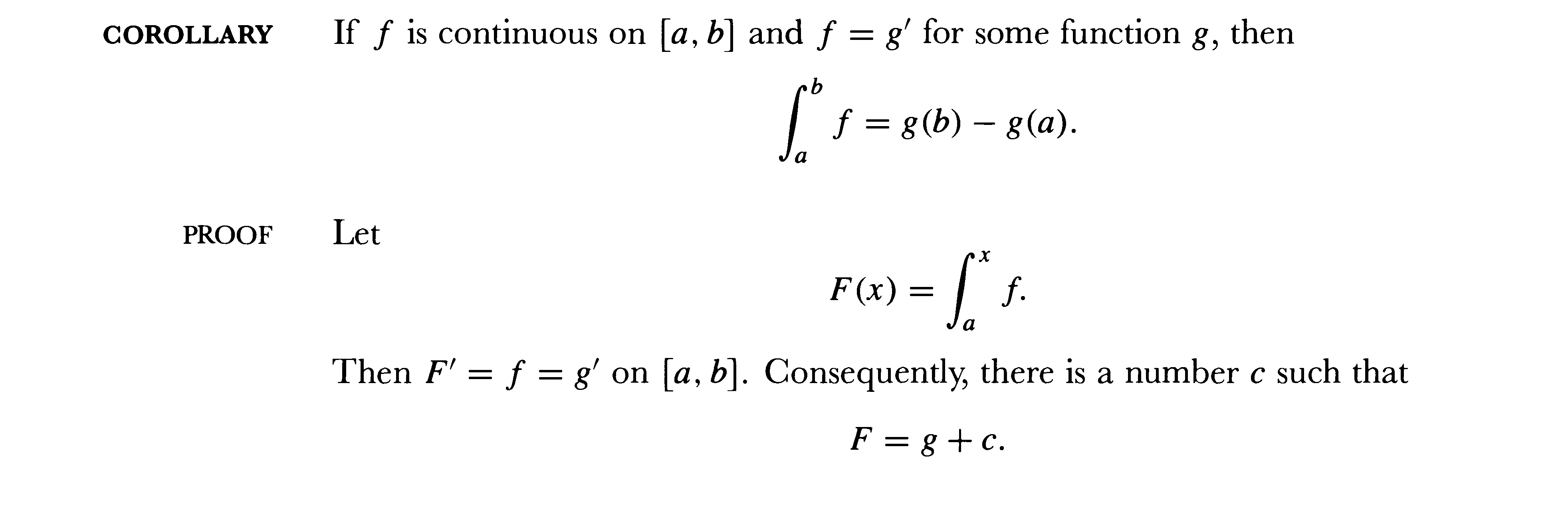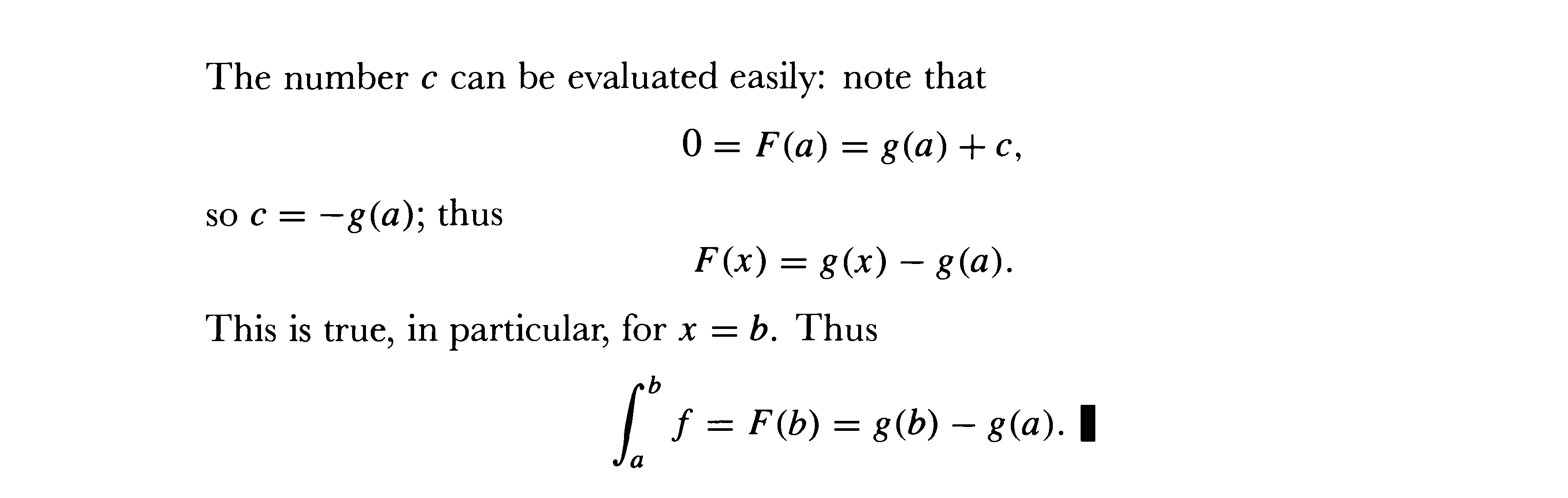Theorem: If f  is continuous on [a,b] then f is integrable on [a,b].

Proof [From Spivak, Calculus, Ch 14]